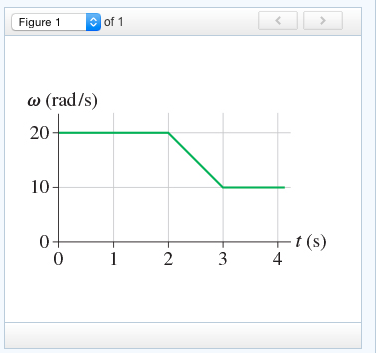# How many revolutions does the object make during the first 4 s?

(Figure 1) shows the angular-velocity-versus-time graph for a
particle moving in a circle. How many revolutions does the object
make during the first 3.3 s ?Figure 1of 1 Figure 1 D of 1 ω (rad/s) 20 10 r (s) 4.

total angular distance = 20*2 + 10*1 + (0.5)*10*1 + 10*0.3 = 58